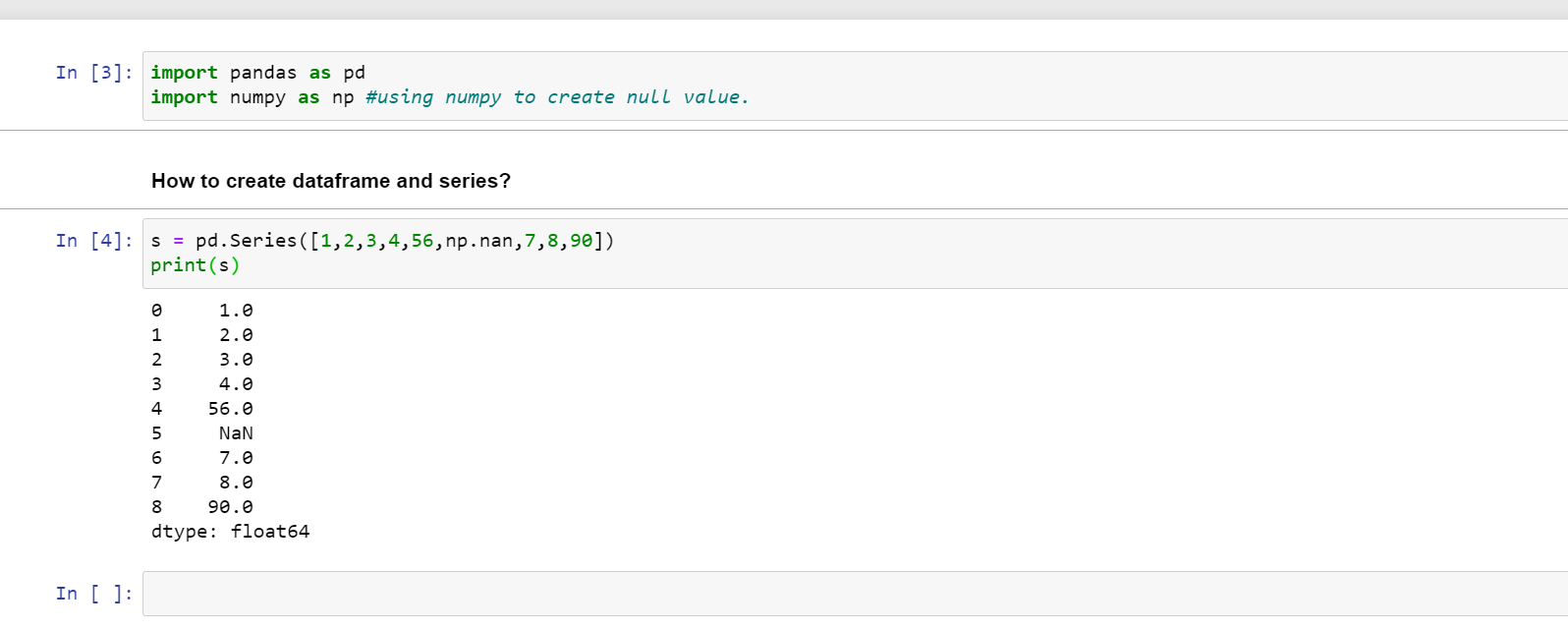1680064740

# 将列值转换为 Pandas 中的字符串

Pandas DataFrame 只是一个二维数据结构或二维数组，以行和列的形式表示数据。换句话说，它与用于存储数据的矩形网格相比。它是开源的，功能强大，快速且易于使用。基本上，在处理大数据时，我们需要分析、操作和更新它们，而 pandas 的图书馆在这方面起着主导作用。我们可以在 的帮助下检查 Pandas 列的类型df.dtypes。基本上，列值由对象、整数等组成。但我们可以将它们转换为字符串。有几种方法可以执行此操作。比如df.astype()- ,casting等。在本文中，我们将探索它们并了解如何在 Pandas 中将列值转换为字符串。让我们创建一个简单的 Pandas DataFrame 并在下面的部分首先检查它的类型：

``````import pandas as pd

student_df = pd.DataFrame({'Name' : ['Alex', 'Rohit', 'Cole', 'Deven', 'John'],
'Marks' : [72, 83, 68, 90, 88]
})

print(student_df)
print(student_df.dtypes)

# Output:
#     Name  Marks
# 0   Alex     72
# 1  Rohit     83
# 2   Cole     68
# 3  Deven     90
# 4   John     88

# Name     object
# Marks     int64
# dtype: object``````

### 示例一：使用将列值转换为字符串astype

``````import pandas as pd

student_df = pd.DataFrame({'Name' : ['Alex', 'Rohit', 'Cole', 'Deven', 'John'],
'Marks' : [72, 83, 68, 90, 88]
})

student_df['Name'] = student_df['Name'].astype('string')
student_df['Marks'] = student_df['Marks'].astype('string')
print(student_df.dtypes)

# Output:
# Name     string
# Marks    string
# dtype: object``````

### 示例二：使用将列值转换为字符串casting

``````import pandas as pd

student_df = pd.DataFrame({'Name' : ['Alex', 'Rohit', 'Cole', 'Deven', 'John'],
'Marks' : [72, 83, 68, 90, 88]
})

casted_df = student_df.astype({'Name':'string', 'Marks':'int32'})
print(casted_df.dtypes)
# Output:
# Name     string
# Marks     int32
# dtype: object``````

## Buddha Community1586702221

## Python Pandas Objects - Pandas Series and Pandas Dataframe

In this post, we will learn about pandas’ data structures/objects. Pandas provide two type of data structures:-

### Pandas Series

Pandas Series is a one dimensional indexed data, which can hold datatypes like integer, string, boolean, float, python object etc. A Pandas Series can hold only one data type at a time. The axis label of the data is called the index of the series. The labels need not to be unique but must be a hashable type. The index of the series can be integer, string and even time-series data. In general, Pandas Series is nothing but a column of an excel sheet with row index being the index of the series.

### Pandas Dataframe

Pandas dataframe is a primary data structure of pandas. Pandas dataframe is a two-dimensional size mutable array with both flexible row indices and flexible column names. In general, it is just like an excel sheet or SQL table. It can also be seen as a python’s dict-like container for series objects.

#python #python-pandas #pandas-dataframe #pandas-series #pandas-tutorial1602550800

## Pandas in Python

Pandas is used for data manipulation, analysis and cleaning.

What are Data Frames and Series?

Dataframe is a two dimensional, size mutable, potentially heterogeneous tabular data.

It contains rows and columns, arithmetic operations can be applied on both rows and columns.

Series is a one dimensional label array capable of holding data of any type. It can be integer, float, string, python objects etc. Panda series is nothing but a column in an excel sheet.

### How to create dataframe and series?

s = pd.Series([1,2,3,4,56,np.nan,7,8,90])

print(s)How to create a dataframe by passing a numpy array?

1. d= pd.date_range(‘20200809’,periods=15)
2. print(d)
3. df = pd.DataFrame(np.random.randn(15,4), index= d, columns = [‘A’,’B’,’C’,’D’])
4. print(df)

#pandas-series #pandas #pandas-in-python #pandas-dataframe #python1616050935

## WORKING WITH GROUPBY IN PANDAS

In my last post, I mentioned the groupby technique  in Pandas library. After creating a groupby object, it is limited to make calculations on grouped data using groupby’s own functions. For example, in the last lesson, we were able to use a few functions such as mean or sum on the object we created with groupby. But with the aggregate () method, we can use both the functions we have written and the methods used with groupby. I will show how to work with groupby in this post.

#pandas-groupby #python-pandas #pandas #data-preprocessing #pandas-tutorial1616395265

## Reading and Writing Data in Pandas

In my last post, I mentioned summarizing and computing descriptive statistics  using the Pandas library. To work with data in Pandas, it is necessary to load the data set first. Reading the data set is one of the important stages of data analysis. In this post, I will talk about reading and writing data.

Before starting the topic, our Medium page includes posts on data science, artificial intelligence, machine learning, and deep learning. Please don’t forget to follow us on Medium 🌱 to see these posts and the latest posts.

Let’s get started.1616395265

## Reading and Writing Data in Pandas

In my last post, I mentioned summarizing and computing descriptive statistics  using the Pandas library. To work with data in Pandas, it is necessary to load the data set first. Reading the data set is one of the important stages of data analysis. In this post, I will talk about reading and writing data.

Before starting the topic, our Medium page includes posts on data science, artificial intelligence, machine learning, and deep learning. Please don’t forget to follow us on Medium 🌱 to see these posts and the latest posts.

Let’s get started.